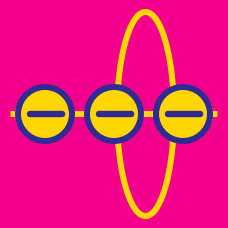Electricity and Magnetism

# Field lines and field strength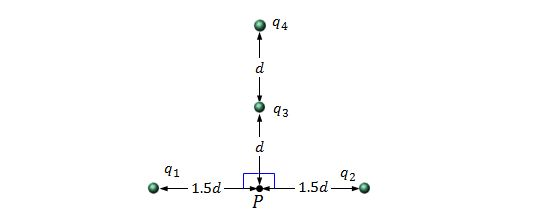Four charged particles are held on the same plane, as shown in the above figure. The respective charges of the particles are $$q_1=q_2=+5.0 \,\mu\text{C},$$ $$q_3=+4.0 \,\mu\text{C},$$ and $$q_4=-16.0 \,\mu\text{C},$$ and the distance $$d$$ in the figure is $$8.0 \text{ mm}.$$ What is the magnitude of the net electric field at point $$P$$ due to the four particles?

The value of electrostatic constant is $$\displaystyle k=\frac{1}{4\pi\varepsilon_0}=8.99 \times 10^9 \text{ N}\cdot\text{m}^2\text{/C}^2.$$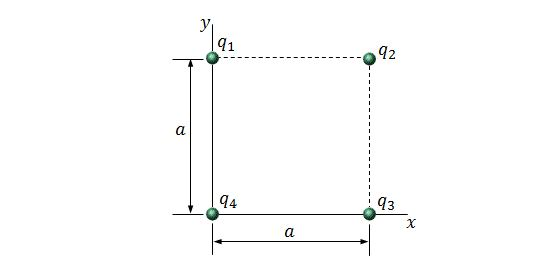Four particles are at the corners of a square with side length $$a=5.00\text{ cm},$$ as shown in the above figure. The respective charges of the particles are $$q_1=+12.0\text{ nC},$$ $$q_2=-24.0\text{ nC},$$ $$q_3=+24.0\text{ nC},$$ and $$q_4=-12.0\text{ nC}.$$ What are the magnitude and direction of the net electric field at the center of the square?

The value of electrostatic constant is $$\displaystyle k=\frac{1}{4\pi\varepsilon_0}=8.99 \times 10^9 \text{ N}\cdot\text{m}^2\text{/C}^2.$$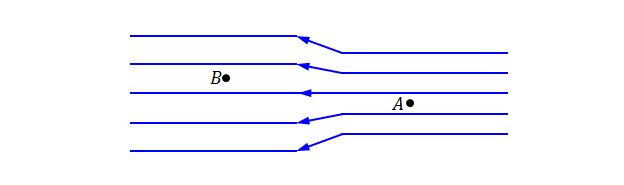In the above figure, the separation of electric field lines on the left is twice the separation of those on the right. If the magnitude of the field at position A is $$70\text{ N/C},$$ what is the magnitude of the field at position B?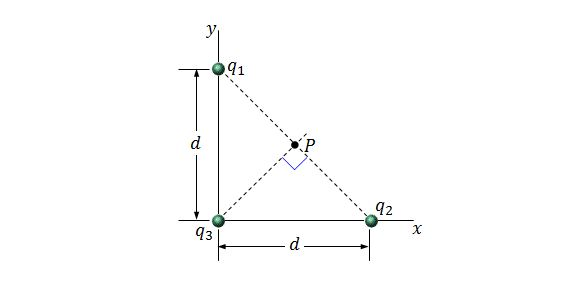Three charged particles are held on the $$xy$$-plane, as shown in the above figure. The distance denoted $$d$$ in the figure is $$d=6.00\,\mu\text{m}.$$ If the respective charges of the particles are $$q_1=q_2=+4e$$ and $$q_3=+8e,$$ what is the magnitude of the net electric field at point $$P$$ due to the three charged particles?

The value of electrostatic constant is $$\displaystyle k=\frac{1}{4\pi\varepsilon_0}=8.99 \times 10^9 \text{ N}\cdot\text{m}^2\text{/C}^2$$ and the elementary charge is $$e=1.60 \times 10^{-19}\text{ C}.$$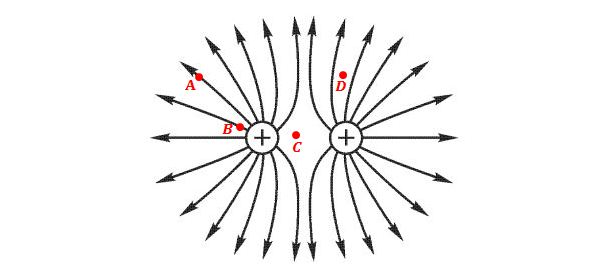The figure above portrays the electric field produced by two positive-charged particles. Which of the following correctly lists the points A, B, C, and D in order of increasing electric field strength?

×

Problem Loading...

Note Loading...

Set Loading...